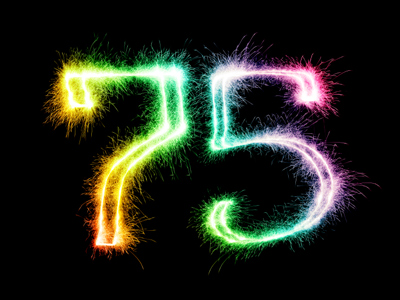If you round 75 to the nearest ten you get 80. Fives are rounded up, not down.

# Rounding Numbers 2 (Medium)

Welcome to this, the second of our Medium level Eleven Plus maths quizzes on Rounding Numbers. If you’ve played our previous quizzes then you should be quite familiar with rounding numbers by now. In this quiz we’ll test you on rounding decimals and ten thousands, along with some easier questions.

Rounding (whether decimals or whole numbers) is all about finding the closest one, ten, hundred, thousand or ten thousand. Is the number you want to round closer to the higher value or the lower one? If it’s the higher value then round up – if it’s the lower value then round down.

One more thing – remember this rule: 0.5s, 5s, 50s, 500s and 5,000s are rounded UP, not DOWN.

And now the quiz. Make sure you read each question properly before you pick your answer. We don’t want to get our tens and hundreds mixed up now do we?

1.
What is 7.493 rounded to the nearest whole number?
10
8
7
6
0.493 is closer to 0 than to 1, so you round downwards
2.
What is 75,396 rounded to the nearest 10?
75,390
75,000
75,400
76,000
6 is closer to 10 than to 0, so you round upwards
3.
What is 13,483 rounded to the nearest 10?
13,500
13,490
13,400
13,480
3 is closer to 0 than to 10, so you round downwards
4.
What is 17,360 rounded to the nearest 100?
17,360
17,400
17,350
17,300
60 is closer to 100 than to 0, so you round upwards
5.
What is 6.5 rounded to the nearest whole number?
7
6
10
0
Although 0.5 is equidistant from 0 and 1, the rule means that you round upwards
6.
What is 777 rounded to the nearest 100?
780
700
770
800
77 is closer to 100 than to 0, so you round upwards
7.
What is 483,636 rounded to the nearest 1,000?
484,000
490,000
483,000
480,000
636 is closer to 1,000 than to 0, so you round upwards
8.
What is 726,385 rounded to the nearest 10,000?
720,000
726,000
730,000
727,000
6,385 is closer to 10,000 than to 0, so you round upwards
9.
What is 374,999 rounded to the nearest 10,000?
375,000
370,000
374,000
380,000
4,999 is closer to 0 than to 10,000, so you round downwards
10.
What is 98,506 rounded to the nearest 1,000?
99,000
98,500
98,000
98,600
506 is closer to 1,000 than to 0, so you round upwards
Author:  Frank Evans